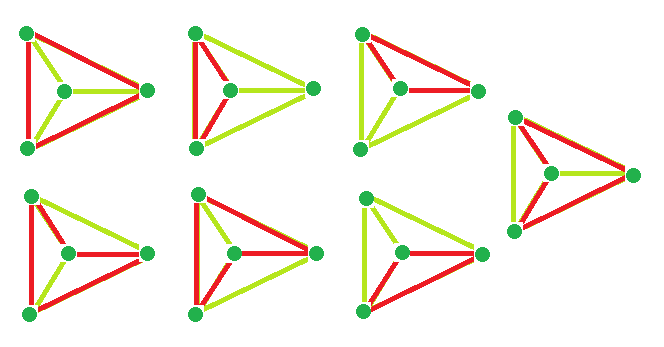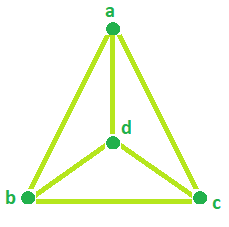# Program to find the diameter, cycles and edges of a Wheel Graph

• Last Updated : 05 Sep, 2022

Wheel Graph: A Wheel graph is a graph formed by connecting a single universal vertex to all vertices of a cycle.

Properties:

• Wheel graphs are Planar graphs.
• There is always a Hamiltonian cycle in the Wheel graph.
• Chromatic Number is 3 and 4, if n is odd and even respectively.

Problem Statement:

Given the Number of Vertices in a Wheel Graph. The task is to find:

1. The Number of Cycles in the Wheel Graph.
2. A number of edges in Wheel Graph.
3. The diameter of a Wheel Graph.

Examples:

```Input: vertices = 4
Output: Number of cycle = 7
Number of edge = 6
Diameter = 1

Input: vertices = 6
Output: Number of cycle = 21
Number of edge = 10
Diameter = 2```

## Recommended: Please try your approach on {IDE} first, before moving on to the solution.

Example #1: For vertices = 4 Wheel Graph, total cycle is 7Example #2:  For vertices = 5 and 7 Wheel Graph Number of edges = 8 and 12 respectively:Example #3: For vertices = 4, the Diameter is 1 as We can go from any vertices to any vertices by covering only 1 edge.Formula to calculate the cycles, edges and diameter:

```Number of Cycle = (vertices * vertices) - (3 * vertices) + 3
Number of edge = 2 * (vertices - 1)
Diameter = if vertices = 4, Diameter = 1
if vertices > 4, Diameter = 2```

Below is the required implementation:

## C++

 `// C++ Program to find the diameter, ` `// cycles and edges of a Wheel Graph` `#include ` `using` `namespace` `std;`   `// Function that calculates the` `// Number of Cycle in Wheel Graph.` `int` `totalCycle(``int` `vertices)` `{` `    ``int` `result = 0;`   `    ``// calculates no. of Cycle.` `    ``result = ``pow``(vertices, 2) - (3 * vertices) + 3;`   `    ``return` `result;` `}`   `// Function that calculates the` `// Number of Edges in Wheel graph.` `int` `Edges(``int` `vertices)` `{` `    ``int` `result = 0;`   `    ``result = 2 * (vertices - 1);`   `    ``return` `result;` `}`   `// Function that calculates the` `// Diameter in Wheel Graph.` `int` `Diameter(``int` `vertices)` `{` `    ``int` `result = 0;`   `    ``// calculates Diameter.` `    ``if` `(vertices == 4)` `        ``result = 1;` `    ``else` `        ``result = 2;`   `    ``return` `result;` `}`   `// Driver Code` `int` `main()` `{` `    ``int` `vertices = 4;`   `    ``cout << ``"Number of Cycle = "` `<< totalCycle(vertices) << endl;` `    ``cout << ``"Number of Edges = "` `<< Edges(vertices) << endl;` `    ``cout << ``"Diameter = "` `<< Diameter(vertices);`   `    ``return` `0;` `}`

## Java

 `//Java Program to find the diameter, ` `// cycles and edges of a Wheel Graph` `import` `java.io.*;`   `class` `GFG` `{` `    ``// Function that calculates the ` `    ``// Number of Cycle in Wheel Graph. ` `    ``static` `int` `totalCycle(``double` `vertices) ` `    ``{ ` `        ``double` `result = ``0``;` `        ``int` `result1 = ``0``;` `      `  `        ``// calculates no. of Cycle. ` `        ``result = Math.pow(vertices, ``2``) - (``3` `* vertices) + ``3``; ` `      `  `        ``result1 = (``int``)(result);` `        ``return` `result1; ` `    ``} ` `      `  `    ``// Function that calculates the ` `    ``// Number of Edges in Wheel graph. ` `    ``static` `int` `Edges(``int` `vertices) ` `    ``{ ` `        ``int` `result = ``0``; ` `      `  `        ``result = ``2` `* (vertices - ``1``); ` `      `  `        ``return` `result; ` `    ``} ` `      `  `    ``// Function that calculates the ` `    ``// Diameter in Wheel Graph. ` `    ``static` `int` `Diameter(``int` `vertices) ` `    ``{ ` `        ``int` `result = ``0``; ` `      `  `        ``// calculates Diameter. ` `        ``if` `(vertices == ``4``) ` `            ``result = ``1``; ` `        ``else` `            ``result = ``2``; ` `      `  `        ``return` `result; ` `    ``}` `    `  `    ``//Driver Code` `    ``public` `static` `void` `main(String[] args)` `    ``{` `        ``int` `vertices = ``4``;` `        `  `        ``System.out.println(``"Number of Cycle = "` `+ totalCycle(vertices));` `        ``System.out.println(``"Number of Edges = "` `+ Edges(vertices));` `        ``System.out.println(``"Diameter = "` `+ Diameter(vertices));` `    ``}` `}`

## Python3

 `# Python3 Program to find the diameter, ` `# cycles and edges of a Wheel Graph `   `# Function that calculates the ` `# Number of Cycle in Wheel Graph. ` `def` `totalCycle(vertices): `   `    ``result ``=` `0`   `    ``# calculates no. of Cycle. ` `    ``result ``=` `(``pow``(vertices, ``2``) ``-` `             ``(``3` `*` `vertices) ``+` `3``)` `    ``return` `result `   `# Function that calculates the ` `# Number of Edges in Wheel graph. ` `def` `Edges(vertices): `   `    ``result ``=` `0` `    ``result ``=` `2` `*` `(vertices ``-` `1``) ` `    ``return` `result `   `# Function that calculates the ` `# Diameter in Wheel Graph. ` `def` `Diameter(vertices): `   `    ``result ``=` `0`   `    ``# calculates Diameter. ` `    ``if` `vertices ``=``=` `4``: ` `        ``result ``=` `1` `    ``else``:` `        ``result ``=` `2`   `    ``return` `result `   `# Driver Code ` `if` `__name__ ``=``=` `"__main__"``:`   `    ``vertices ``=` `4`   `    ``print``(``"Number of Cycle ="``, ` `           ``totalCycle(vertices)) ` `    ``print``(``"Number of Edges ="``, Edges(vertices)) ` `    ``print``(``"Diameter ="``, Diameter(vertices)) `   `# This code is contributed by Rituraj Jain`

## C#

 `// C# Program to find the diameter, ` `// cycles and edges of a Wheel Graph` `using` `System;` `class` `GFG` `{` `// Function that calculates the ` `// Number of Cycle in Wheel Graph. ` `static` `int` `totalCycle(``double` `vertices) ` `{ ` `    ``double` `result = 0;` `    ``int` `result1 = 0;`   `    ``// calculates no. of Cycle. ` `    ``result = Math.Pow(vertices, 2) -` `                     ``(3 * vertices) + 3; `   `    ``result1 = (``int``)(result);` `    ``return` `result1; ` `} `   `// Function that calculates the ` `// Number of Edges in Wheel graph. ` `static` `int` `Edges(``int` `vertices) ` `{ ` `    ``int` `result = 0; `   `    ``result = 2 * (vertices - 1); `   `    ``return` `result; ` `} `   `// Function that calculates the ` `// Diameter in Wheel Graph. ` `static` `int` `Diameter(``int` `vertices) ` `{ ` `    ``int` `result = 0; `   `    ``// calculates Diameter. ` `    ``if` `(vertices == 4) ` `        ``result = 1; ` `    ``else` `        ``result = 2; `   `    ``return` `result; ` `}`   `// Driver Code` `public` `static` `void` `Main()` `{` `    ``int` `vertices = 4;` `    `  `    ``Console.WriteLine(``"Number of Cycle = "` `+ ` `                      ``totalCycle(vertices));` `    ``Console.WriteLine(``"Number of Edges = "` `+ ` `                           ``Edges(vertices));` `    ``Console.WriteLine(``"Diameter = "` `+ ` `                       ``Diameter(vertices));` `}` `}`   `// This code is contributed by inder_verma`

## PHP

 ``

Output

```Number of Cycle = 7
Number of Edges = 6
Diameter = 1```

My Personal Notes arrow_drop_up
Recommended Articles
Page :